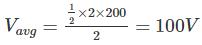Courses

# Electrical Circuits 1 MCQ

## 20 Questions MCQ Test Mock Test Series for SSC JE Electrical Engineering (Hindi) | Electrical Circuits 1 MCQ

Description
This mock test of Electrical Circuits 1 MCQ for Electrical Engineering (EE) helps you for every Electrical Engineering (EE) entrance exam. This contains 20 Multiple Choice Questions for Electrical Engineering (EE) Electrical Circuits 1 MCQ (mcq) to study with solutions a complete question bank. The solved questions answers in this Electrical Circuits 1 MCQ quiz give you a good mix of easy questions and tough questions. Electrical Engineering (EE) students definitely take this Electrical Circuits 1 MCQ exercise for a better result in the exam. You can find other Electrical Circuits 1 MCQ extra questions, long questions & short questions for Electrical Engineering (EE) on EduRev as well by searching above.
QUESTION: 1

### Three wires having conductance’s 2, 3 and 6 mho respectively are connected in parallel in a circuit. The equivalent resistance in the circuit will be:

Solution:

Given that,

G1 = 2 mho

G2 = 3 mho

G3 = 6 mho

These are connected in parallel.

Geq = G1 + G2 + G3 + …… + Gn

⇒ Geq = 2 + 3 + 6 = 11 mho

QUESTION: 2

### What is the difference between an atom and an ion?

Solution:

An ion is a particle or collection of particles with a net positive or negative charge.

An atom is the basic unit of an element. The identity of an element is determined by the number of positively charged protons in the atom’s nucleus. A stable atom contains the same number of electrons as protons and no net charge.

QUESTION: 3

### Two heaters rated at 1000 W, 250V each are connected in series across a 250 V, 50 Hz ac mains. The total power drawn from the supply would be _____ watt.

Solution: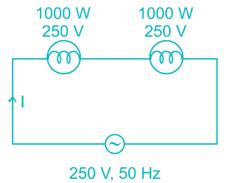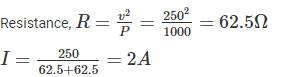Total power drawn from the supply

P = VI = 250 × 2 = 500 W.

QUESTION: 4

Which of the following does not have dual relationship?

Solution: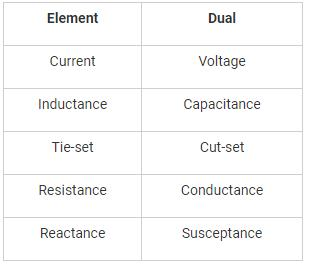QUESTION: 5

If each branch of star connection is 3 Ω. Then its corresponding delta connection will contain ______.

Solution: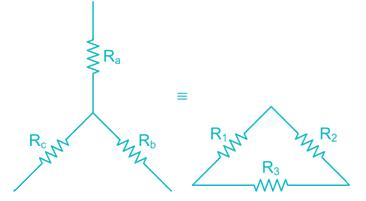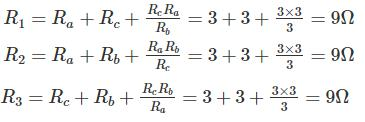QUESTION: 6

Find out resistance between X and Y in following: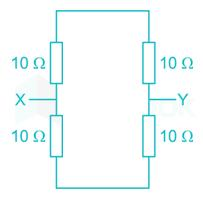Solution: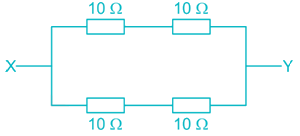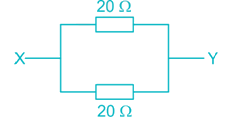QUESTION: 7

In a four-branch parallel circuit, there are 10 mA of current in each branch. If one of the branches is open, the current in each of the other three branches is:

Solution:

All the four branches are connected in parallel, so the voltage across every branch is same. If one of the branch is open, it doesn’t affect the other branches current. Because the voltage and impedance across remaining branches will remain same.

QUESTION: 8

The number of mesh currents required in the circuit given below is: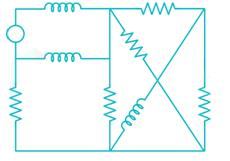Solution:

In the given network

Number of branches (b) = 9

Number of nodes (n) = 5

Number of mesh currents = b – n + 1

= 9 – 5 + 1 = 5

QUESTION: 9

Four resistors of 50 Ω, 50 Ω, 100 Ω and 100 Ω each are connected in parallel across 100 V dc supply. What is the current though each branch if both the resistors of 50 Ω burn out?

Solution: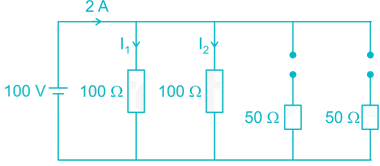Current flowing through 100 Ω resistance,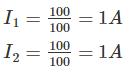QUESTION: 10

A current source and a voltage source are connected in parallel as shown below. Suppose that vs = 12 V and is = 3 A.What is the power supplied by each source?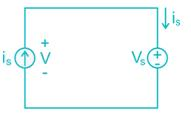Solution: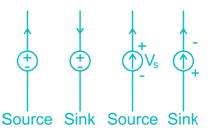Power supplied by current source = 12 × 3 = 36 W

Here current source acts as source

Power supplied by voltage source = 12 × (-3) = -36W

Here voltage source acts as sink.

QUESTION: 11

A voltage source of 10 V and resistor are connected in series. Specify the resistance R so that both of the following conditions are satisfied:

i > 40 mA and the power absorbed by the resistors is < 0.5 W.

Solution: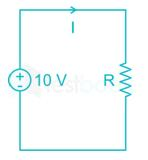Given that,

I > 40 mA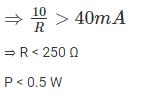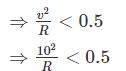⇒ R > 200

⇒ 200 < R < 250

QUESTION: 12

Determine the value of the current i for the circuit shown below.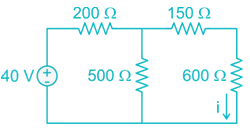Solution: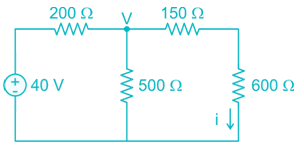Apply nodal analysis at node v.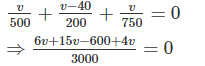⇒ 25V = 600

⇒ v = 24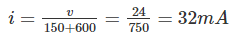QUESTION: 13

An equivalent single current source between ‘A’ and ‘B’ in the following figure will be: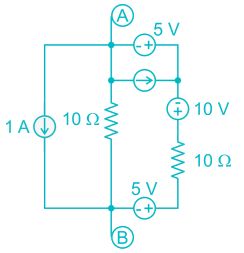Solution:

An equivalent single current source is nothing but Norton’s equivalent

To find short circuit current (IN)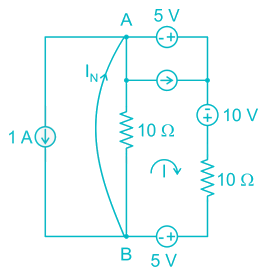Apply kVL

-5 – 10 + 10 I + 5 = 0

⇒ I = 1A

Apply kCL at point B,

IN = 1 + 1 = 2A

To find Norton’s resistance, we need to short circuit the voltage sources and open circuit the current sources.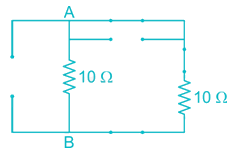RAB = (10 || 10) = 5Ω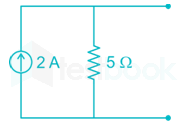QUESTION: 14

In the interconnection of ideal sources shown in the following figure, it is known that the 60 V source is absorbing power. Which of the following can be the value of the current source i?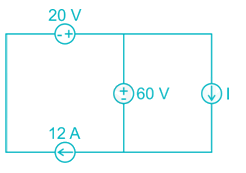Solution: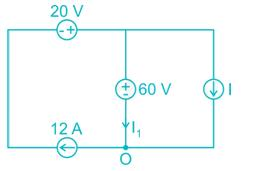kCL at O

⇒ I1 + I = 12

⇒ I = 12 – I1

By the above equation,

I should be less than 12 A.

Hence 10 A can be the value of the current source I.

QUESTION: 15

The capacitor voltage in the circuit long after the switch has been closed is: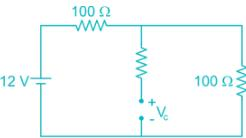Solution:

After t = 0, at steady state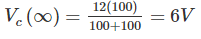QUESTION: 16

A sinusoidal current is given as i(t) = 150 cos (1000πt – 45°)mA. Determine the period T and time t2 at which the first positive peak occurs:

Solution:

i(t) = 150 cos (1000 πt – 45°) mA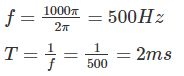i(t) can attain max at

1000πt - π/4 = 0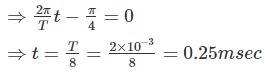QUESTION: 17

In a series RL circuit 5 A current is flowing, voltage drop across R is 16 V and voltage drop across L is 12 V calculate impedance –

Solution: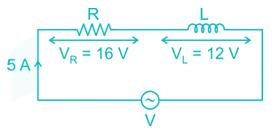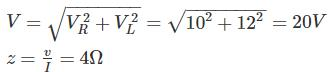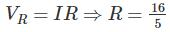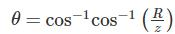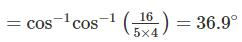⇒ z = 4 ∠36 - 9°

QUESTION: 18

The current i(t), through a 10 Ω resistor in series with an inductance is given by I(t) = 3 + 4 sin(100t + 450) + 4sin(300t + 600) amperes the RMS values of the current and power dissipation in the circuit are

Solution: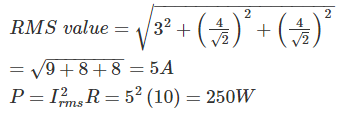QUESTION: 19

A series RLC circuit has Q of 100 and an impendence of (100 ± j0)Ω at its resonant angular frequency of 107 rad/s. The value of R and L respectively –

Solution:

Q = 100

R = 100 Ω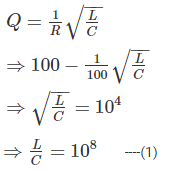for resonance condition,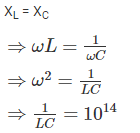⇒ LC = 10-14      ----(2)

By (1) and (2)

⇒ L = 10-3 H

QUESTION: 20

What will be the average value of the sawtooth waveform shown below?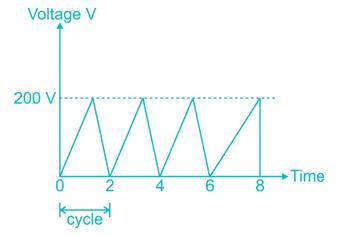Solution: